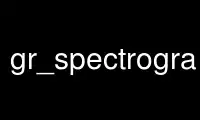# gr_spectrogram_plot_c - Online in the CloudThis is the command gr_spectrogram_plot_c that can be run in the OnWorks free hosting provider using one of our multiple free online workstations such as Ubuntu Online, Fedora Online, Windows online emulator or MAC OS online emulator

### PROGRAM:

#### NAME

gr_spectrogram_plot - gnuradio spectrogram plotting application

#### SYNOPSIS

gr_spectrogram_plot_c [options]

#### DESCRIPTION

Plots the spectrogram (waterfall) of a list of files. Files are a binary list of complex
floats.

#### OPTIONS

-h, --help
show this help message and exit

-N NSAMPLES, --nsamples=NSAMPLES
Set the number of samples to display [default=1000000]

-S START, --start=START
Starting sample number [default=0]

-L PSD_SIZE, --psd-size=PSD_SIZE
Set the FFT size of the PSD [default=2048]

-f CENTER_FREQUENCY, --center-frequency=CENTER_FREQUENCY
Set the center frequency of the signal [default=0.0]

-r SAMPLE_RATE, --sample-rate=SAMPLE_RATE
Set the sample rate of the signal [default=1.0]

-a AVERAGE, --average=AVERAGE
Set amount of averaging (smaller=more averaging) [default=1.0]

gr_spectrogram_plot_c: error: no such option: --version

Use gr_spectrogram_plot_c online using onworks.net services